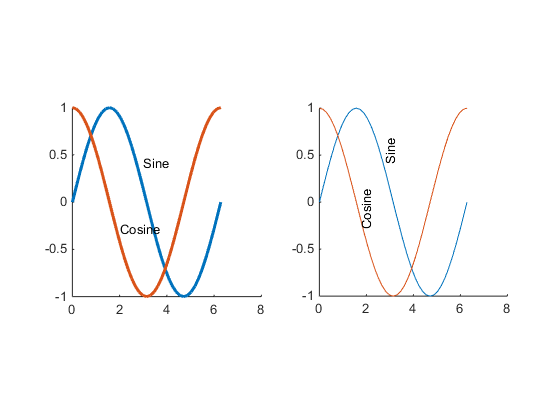## 多级默认值

```t = 0:pi/20:2*pi; s = sin(t); c = cos(t); figure('defaultAxesPlotBoxAspectRatio',[1 1 1],... 'defaultAxesPlotBoxAspectRatioMode','manual'); subplot(1,2,1,'defaultLineLineWidth',2); hold on plot(t,s,t,c) text('Position',[3 0.4],'String','Sine') text('Position',[2 -0.3],'String','Cosine') subplot(1,2,2,'defaultTextRotation',90); hold on plot(t,s,t,c) text('Position',[3 0.4],'String','Sine') text('Position',[2 -0.3],'String','Cosine')```